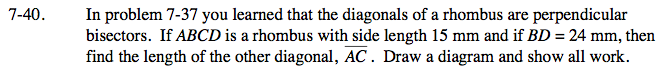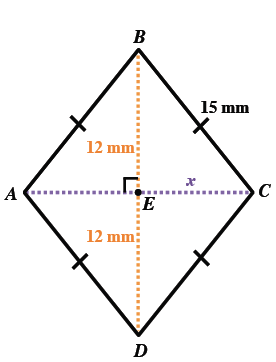### Home > GC > Chapter 7 > Lesson 7.1.4 > Problem7-40

7-40.

In problem 7-37 you learned that the diagonals of a rhombus are perpendicular bisectors. If ABCD is a rhombus with side length 15 mm and if BD = 24 mm, then find the length of the other diagonal,.Draw a diagram and show all work. Homework Help ✎Make an accurate and well-labeled diagram of the situation.

Use the right triangle ΔBCE and the Pythagorean Theorem to solve for x.

x = 18 mm